Home > A2C > Chapter 8 > Lesson 8.2.1 > Problem8-121

8-121.
1. Evaluate each of the following expressions exactly. Homework Help ✎

1.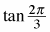2.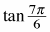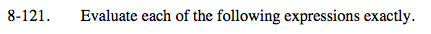Draw a unit circle and locate each angle. Draw a reference triangle. Use special triangles.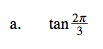$-\sqrt{3}$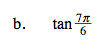$\frac{\sqrt{3}}{3}$